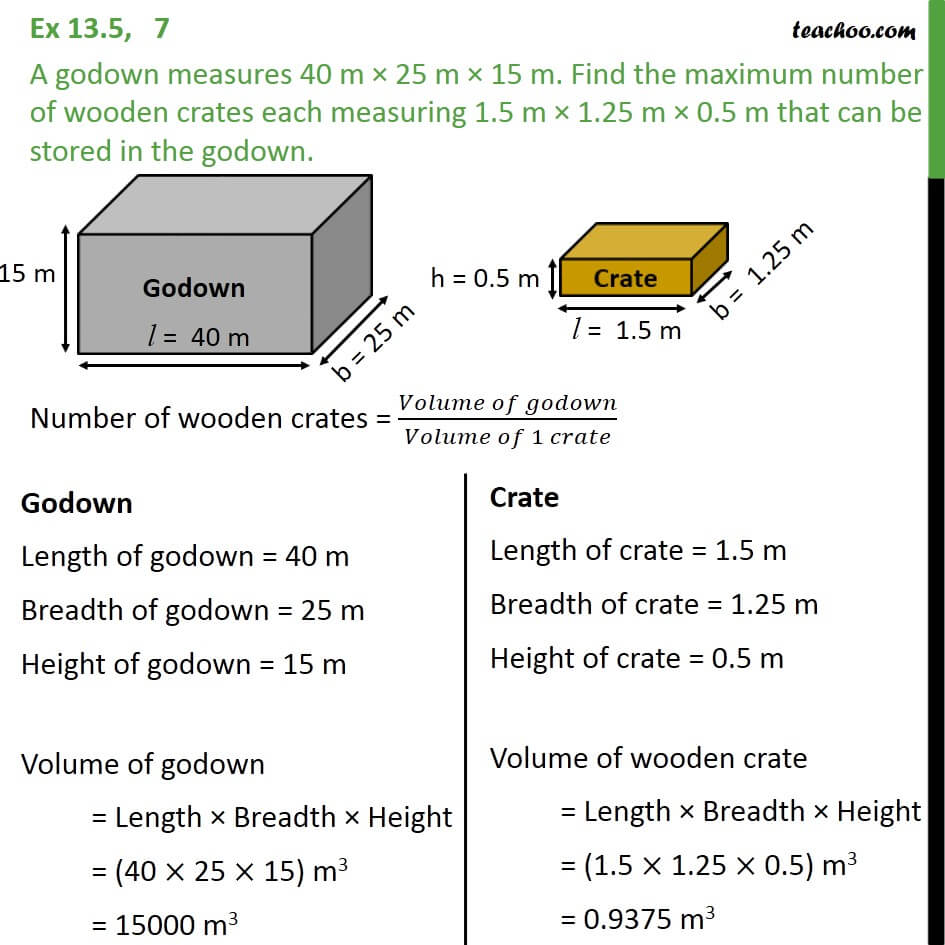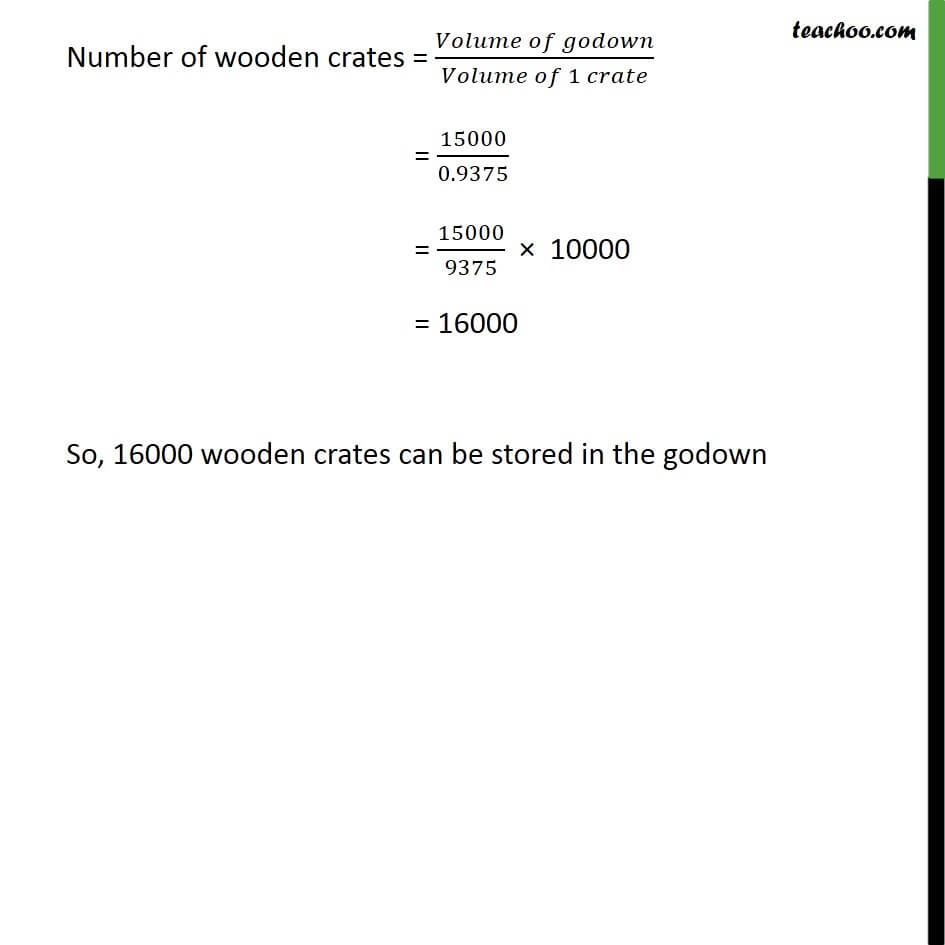Volume of Cube and Cuboid

Chapter 11 Class 9 Surface Areas and Volumes
Serial order wiseThe calculation in this video is wrong, we have mistakenly taken height of godown as 10, but it is 15. 1

Learn in your speed, with individual attention - Teachoo Maths 1-on-1 Class

### Transcript

Question 7 A godown measures 40 m × 25 m × 10 m. Find the maximum number of wooden crates each measuring 1.5 m × 1.25 m × 0.5 m that can be stored in the godown. Godown Length of godown = 40 m Breadth of godown = 25 m Height of godown = 15 m Volume of godown = Length × Breadth × Height = (40 × 25 × 15) m3 = 15000 m3 Crate Length of crate = 1.5 m Breadth of crate = 1.25 m Height of crate = 0.5 m Volume of wooden crate = Length × Breadth × Height = (1.5 × 1.25 × 0.5) m3 = 0.9375 m3 Number of wooden crates = (𝑉𝑜𝑙𝑢𝑚𝑒 𝑜𝑓 𝑔𝑜𝑑𝑜𝑤𝑛)/(𝑉𝑜𝑙𝑢𝑚𝑒 𝑜𝑓 1 𝑐𝑟𝑎𝑡𝑒) = 15000/0.9375 = 15000/9375 × 10000 = 16000 So, 16000 wooden crates can be stored in the godown